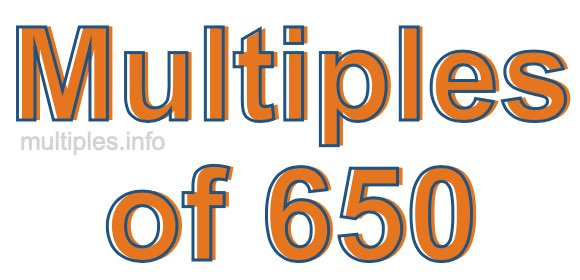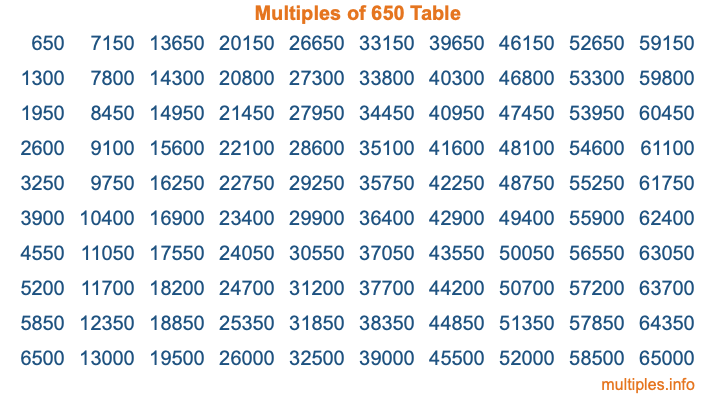Multiples of 650Welcome to the Multiples of 650 page. Here we will first teach you everything you will ever need to know about the multiples of 650, and then give you a study guide summary of everything we taught you to make sure you remember it all. Use this page to look up facts and learn information about the multiples of 650. This page will make you a multiples of six hundred fifty expert!

Definition of Multiples of 650
Multiples of 650 are all the numbers that when divided by 650 equal an integer. Each of the multiples of 650 are called a multiple. A multiple of 650 is created by multiplying 650 by an integer.

Therefore, to create a list of multiples of 650, you start with 1 multiplied by 650, then 2 multiplied by 650, then 3 multiplied by 650, and so on for as long as you want. Thus, the list of the first five multiples of 650 is 650, 1300, 1950, 2600, and 3250. To see a larger list of multiples of 650, see the printable image of Multiples of 650 further down on this page. We also have a category where you can choose any nth multiple of 650.

Multiples of 650 Checker
The Multiples of 650 Checker below checks to see if any number of your choice is a multiple of 650. In other words, it checks to see if there is any number (integer) that when multiplied by 650 will equal your number. To do that, we divide your number by 650. If the the quotient is an integer, then your number is a multiple of 650.

Is  a multiple of 650?

Least Common Multiple of 650 and ...
A Least Common Multiple (LCM) is the lowest multiple that two or more numbers have in common. This is also called the smallest common multiple or lowest common multiple and is useful to know when you are adding our subtracting fractions. Enter one or more numbers below (650 is already entered) to find the LCM.

Check out our LCM Calculator if you need more details about the Least Common Multiple or if you need the LCM for different numbers for adding and subtraction fractions.

nth Multiple of 650
As we stated above, 650 is the first multiple of 650, 1300 is the second multiple of 650, 1950 is the third multiple of 650, and so on. Enter a number below to find the nth multiple of 650.

th multiple of 650

Multiples of 650 vs Factors of 650
650 is a multiple of 650 and a factor of 650, but that is where the similarities end. All postive multiples of 650 are 650 or greater than 650. All positive factors of 650 are 650 or less than 650.

Below is the beginning list of multiples of 650 and the factors of 650 so you can compare:

Multiples of 650: 650, 1300, 1950, 2600, 3250, etc.

Factors of 650: 1, 2, 5, 10, 13, 25, 26, 50, 65, 130, 325, 650

As you can see, the multiples of 650 are all the numbers that you can divide by 650 to get a whole number. The factors of 650, on the other hand, are all the whole numbers that you can multiply by another whole number to get 650.

It's also interesting to note that if a number (x) is a factor of 650, then 650 will also be a multiple of that number (x).

Multiples of 650 vs Divisors of 650
The divisors of 650 are all the integers that 650 can be divided by evenly. Below is a list of the divisors of 650.

Divisors of 650: 1, 2, 5, 10, 13, 25, 26, 50, 65, 130, 325, 650

The interesting thing to note here is that if you take any multiple of 650 and divide it by a divisor of 650, you will see that the quotient is an integer.

Multiples of 650 Table
Below is an image of the first 100 multiples of 650 in a table. The table is in chronological order, column by column. The first column has the first ten multiples of 650, the second column has the next ten multiples of 650, and so on.The Multiples of 650 Table is also referred to as the 650 Times Table or Times Table of 650. You are welcome to print out our table for your studies.

Negative Multiples of 650
Although not often discussed or needed in math, it is worth mentioning that you can make a list of negative multiples of 650 by multiplying 650 by -1, then by -2, then by -3, and so on, to get the following list of negative multiples of 650:

-650, -1300, -1950, -2600, -3250, etc.

Multiples of 650 Summary
Below is a summary of important Multiples of 650 facts that we have discussed on this page. To retain the knowledge on this page, we recommend that you read through the summary and explain to yourself or a study partner why they hold true.

There are an infinite number of multiples of 650.

A multiple of 650 divided by 650 will equal a whole number.

650 divided by a factor of 650 equals a divisor of 650.

The nth multiple of 650 is n times 650.

The largest factor of 650 is equal to the first positive multiple of 650.

650 is a multiple of every factor of 650.

650 is a multiple of 650.

A multiple of 650 divided by a divisor of 650 equals an integer.

650 divided by a divisor of 650 equals a factor of 650.

Any integer times 650 will equal a multiple of 650.

Multiples of a Number
Here you can get the multiples of another number, all with the same attention to detail as we did for multiples of 650 on this page.

Multiples of
Multiples of 651
Did you find our page about multiples of six hundred fifty educational? Do you want more knowledge? Check out the multiples of the next number on our list!